# Coulomb’s Law¶

Coulomb’s law describes the force between two points charges (q2 on q1):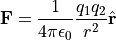where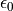is the electric constant, r is the distance between the two points, and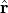is a unit vector pointing in the direction from q2 to q1. Alternately it can be expressed in terms of electric field (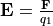):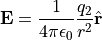Since forces (and electric fields) are additive, Coulomb’s law in theory allows deriving the electric field from any distribution of charge in space. In practice, the Laplace Equation or Poisson Equation are often more useful for solving the electric field (and SIMION Refine uses them for that).

One place where Coulomb’s law does come up in SIMION is in its charge repulsion methods (see SIMION Example: repulsion and Space Charge) to simulate things like ion-ion repulsions, ignoring interactions between ions and electrode surface charges (which the Poisson Equation will fully account for).

Observe that the electric potential from a point charge is proportional to 1/r, as seen by simply integrating Coulomb’s law: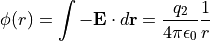Interestingly, a spherical capacitor also has a 1/r potential: The sphere with uniform surface charge generates the same field as a single point charge.